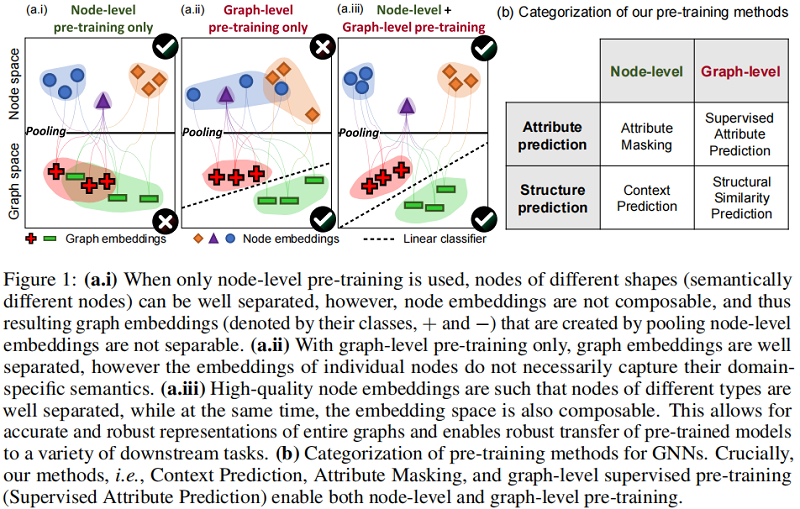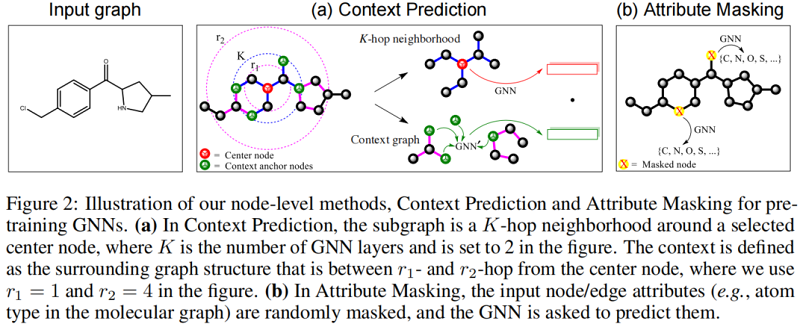# 论文解读《Strategies for Pre-training Graph Neural Networks》

2022-05-29 16:04 3067 查看

# 1 Introduction

预训练的解决的问题：

• [li]首先，特定于任务的标记数据可能极其稀缺；
• 其次，来自真实应用程序的图数据通常包含分布外的样本，这意味着训练集中的图在结构上与测试集中的图非常不同。分布外预测在真实世界的图数据集中很常见；
[/li]

本文的预训练：在节点和整个图的水平上预先训练一个表达性的GNN，以便 GNN 可同时学习有用的局部和全局表示。

• [li]如果仅做节点级别的预训练，虽然不同的节点能够很好地被区分，但节点组合成的图不能被很好地被区分；
• 如果仅做图级别的预训练，虽然不同的图能够被很好地区分，但图中节点的表示不能够被区分；
• 故既需要节点级别，也需图级，这样不同的节点表示和图表示都能够在空间中很好地区分开来；
[/li]在 2.1 节将描述本文的节点级训练方法，在 2.2 节描述图级预训练方法，在 2.3 节描述整个完整的训练方法。

## 2.1 Node-level pre-training

对于 GNN 的节点级预训练，我们的方法是使用易于获取的未标记数据来在图中捕获特定领域的知识/规律。

### 2.1.1 Context prrediction :exploiting distribution of graph structure

在上下文预测中，我们使用子图来预测它们周围的图结构。我们的目标是对GNN进行预训练，使其映射出现在类似结构环境中的节点到附近的嵌入。

Neighborhood and context graphs

对于每个节点 $v$，我们定义 $v$ 的邻域图和上下文图如下：

$\text{K-hop neighborhood}$：$v$ 的 $k$ 跳邻域包含了图中距离 $v$ 最多为 $k$ 跳的所有节点和边。这是由于一个 $k$ 层GNN通过 $v$ 的 $k$ 阶邻域聚集信息，因此节点嵌入 $h_{v}^{(K)}$ 依赖于距离 $v$ 最多 $k$ 跳的节点。

$\text{Context graph}$：我们将节点 $v$ 的上下文图定义为围绕着 $v$ 的邻域的图结构。上下文图由两个超参数 $r_{1}$ 和 $r_{2}$ 描述，它表示一个在 $r_{1}$ 跳和 $r_{2}$ 跳之间的子图(即，它是一个宽度为 $r_{2}-r_{1}$ 的环)。Encoding context into a fixed vector using an auxiliary GNN

为实现上下文预测，我们将上下文图编码为固定长度的向量。为此，我们使用了一个辅助的GNN，我们称之为上 $\text{context GNN}$。如 Figure 2(a) 所示，我们首先应用 $\text{context GNN}$ 来获得上下文图中的节点嵌入。然后，对 context anchor nodes 的嵌入进行平均，以获得固定长度的上下文嵌入。对于图 $G$ 中的节点 $v$，我们将其对应的上下文嵌入表示为 $c_{v}^{G}$。

Learning via negative sampling

我们使用负抽样来共同学习 main GNN 和 context GNN 。main GNN 对邻域进行编码，以获得节点嵌入。context GNN 编码上下文图以获得上下文嵌入。特别是，上下文预测的学习目标是对特定邻域和特定上下文图是否属于同一节点的二分类：

$\sigma\left(h_{v}^{(K) \top} c_{v^{\prime}}^{G^{\prime}}\right) \approx 1\left\{v \text { and } v^{\prime} \text { are the same nodes }\right\}$

我们要么让 $v^{\prime}=v$ 和 $G^{\prime}=G$（即，a positive neighborhood-context pair），要么我们从一个随机选择的图 $G^{\prime}$（即，一个负的邻域上下文对）中随机抽取 $v^{\prime}$。我们使用 $1$ 的负采样比（每一个正对一个负对），并使用负对数似然作为损失函数。经过预训练后，保留 main GNN 作为我们的预训练模型。

Attibute masking :exploiting distribution of graph attributes

属性掩蔽训练前的工作原理如下：我们掩蔽节点/边缘属性，然后让 GNNs 基于邻近结构预测这些属性。Figure 2 (b) 说明了我们提出的方法应用于分子图的方法。具体来说，我们随机掩蔽输入的节点/边缘属性，例如分子图中的原子类型，用特殊的掩蔽指示符替换它们。然后，我们应用GNNs来获得相应的节点/边嵌入（边缘嵌入可以作为边的末端节点的节点嵌入的和来得到）。最后，在嵌入之上应用一个线性模型来预测掩蔽节点/边缘属性。我们应用消息传递，我们处理非全连通图，目的是捕获分布在不同图结构上的节点/边属性的规律。此外，我们允许屏蔽边缘属性，而不是屏蔽节点属性。

我们的节点和边缘属性掩蔽方法特别有利于来自科学领域的丰富注释图。例如，（1）在分子图中，节点属性对应于原子类型，并捕获它们如何在图上分布，使gnn能够学习简单的化学规则，如价，以及可能更复杂的化学现象，如官能团的电子或空间性质。类似地，（2）在蛋白质-蛋白质相互作用(PPI)图中，边缘属性对应于一对蛋白质之间不同类型的相互作用。捕获这些属性如何在PPI图中分布，使gnn能够了解不同的交互作用是如何相互关联和相互关联的。

## 2.2 Graph-level pre-training

我们的目标是预先训练 GNN，以生成有用的图嵌入，由通过第 2.1 节中的方法获得的有意义的节点嵌入。我们的目标是确保节点和图嵌入都是高质量的，这样图嵌入就是健壮的，并且可以在下游任务中进行转移，如 Figure 1 (a.iii) 所示。此外，对于图级的预训练有两个选项，如 Figure 1 (b)所示：making predictions about domain-specifific attributes of entire graphs，或者making predictions about graph structure。

### 2.2.1 Supervised graph-level property prediction

由于图级表示 $h_{G}$ 直接用于下游预测任务的微调，因此需要将特定域的信息直接编码到 $h_{G}$ 中。在这里，我们使用第一种方式并考虑一种实用的方法来预训练图表示；图级多任务监督预训练来联合预测不同的监督图标签集。这类似于ImageNet上预测一组对象类别的大规模多任务监督预训练。具体地说，我们在图表示之上用线性分类器来联合预测图的属性，其中每个属性对应于一个二值分类任务。

## 2.3 Overview :pre-training GNNs and fine-tuning for downstream tasks

总之，我们的预训练策略是先执行节点级自监督预训练（第2.1节），然后执行图形级多任务监督预训练（第2.2节）。当GNN预训练完成后，我们对GNN进行微调，以完成下游任务。具体来说，我们在图级表示的基础上加入随机初始化的线性分类器来预测下游的图标签。整个模型，即预先训练的GNN和下游线性分类器，随后以端到端的方式进行微调。

时间复杂性：所有预训练方法的时间复杂度与边的数量最多是线性的，因此，计算开销很小。此外，当我们动态地转换数据（例如，屏蔽输入节点/边缘特征，采样上下文图）时，几乎没有内存开销。